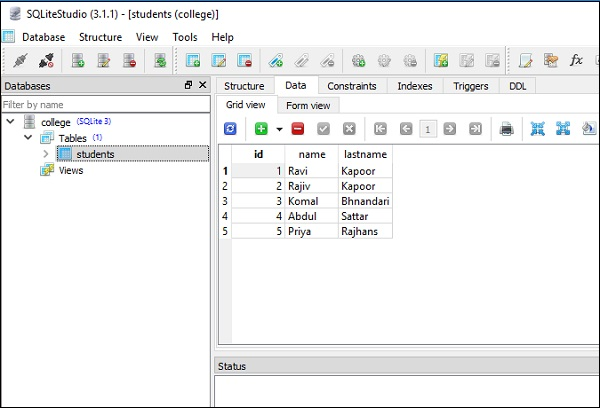# Using UPDATE Expression

The update() method on target table object constructs equivalent UPDATE SQL expression.

```table.update().where(conditions).values(SET expressions)
```

The values() method on the resultant update object is used to specify the SET conditions of the UPDATE. If left as None, the SET conditions are determined from those parameters passed to the statement during the execution and/or compilation of the statement.

The where clause is an Optional expression describing the WHERE condition of the UPDATE statement.

Following code snippet changes value of ‘lastname’ column from ‘Khanna’ to ‘Kapoor’ in students table −

```stmt = students.update().where(students.c.lastname == 'Khanna').values(lastname = 'Kapoor')
```

The stmt object is an update object that translates to −

```'UPDATE students SET lastname = :lastname WHERE students.lastname = :lastname_1'
```

The bound parameter lastname_1 will be substituted when execute() method is invoked. The complete update code is given below −

```from sqlalchemy import create_engine, MetaData, Table, Column, Integer, String
engine = create_engine('sqlite:///college.db', echo = True)

students = Table(
'students',
meta,
Column('id', Integer, primary_key = True),
Column('name', String),
Column('lastname', String),
)

conn = engine.connect()
stmt=students.update().where(students.c.lastname=='Khanna').values(lastname='Kapoor')
conn.execute(stmt)
s = students.select()
conn.execute(s).fetchall()
```

The above code displays following output with second row showing effect of update operation as in the screenshot given −

```[
(1, 'Ravi', 'Kapoor'),
(2, 'Rajiv', 'Kapoor'),
(3, 'Komal', 'Bhandari'),
(4, 'Abdul', 'Sattar'),
(5, 'Priya', 'Rajhans')
]
```Note that similar functionality can also be achieved by using update() function in sqlalchemy.sql.expression module as shown below −

```from sqlalchemy.sql.expression import update
stmt = update(students).where(students.c.lastname == 'Khanna').values(lastname = 'Kapoor')
```